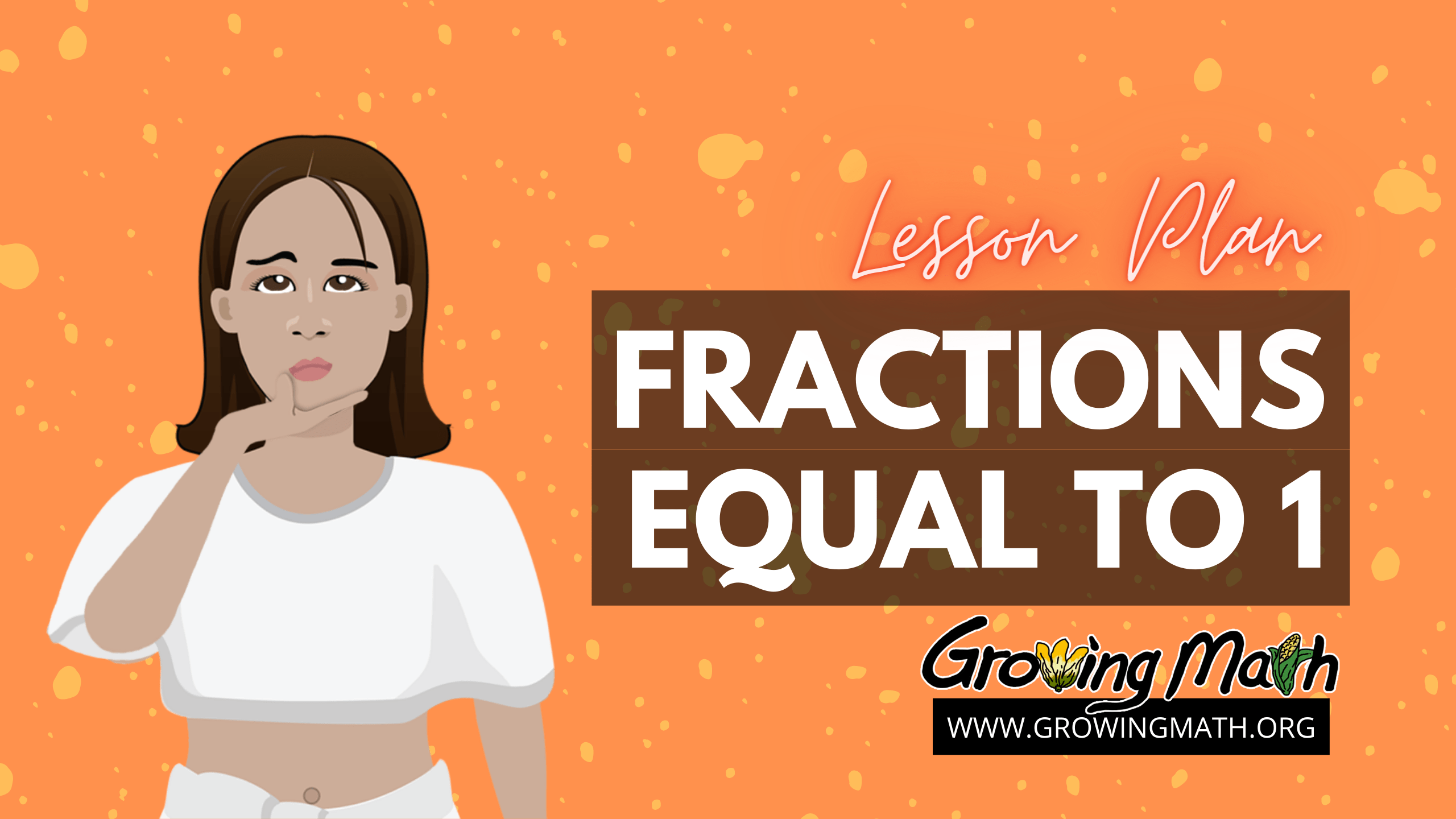# Fractions Equal to 1

## 📖STANDARD

CCSS.MATH.CONTENT.3.NF.A.2 Understand a fraction as a number on the number line; represent fractions on a number line diagram.

CCSS.MATH.CONTENT.3.NF.A.2.A Represent a fraction 1/b on a number line diagram by defining the interval from 0 to 1 as the whole and partitioning it into b equal parts. Recognize that each part has size 1/b and that the endpoint of the part based at 0 locates the number 1/b on the number line.

CCSS.MATH.CONTENT.3.NF.A.2.B Represent a fraction a/b on a number line diagram by marking off a lengths 1/b from 0. Recognize that the resulting interval has size a/b and that its endpoint locates the number a/b on the number line.

CCSS.MATH.CONTENT.4.NF.B.3.D Solve word problems involving addition and subtraction of fractions referring to the same whole and having like denominators, e.g., by using visual fraction models and equations to represent the problem.

45 minutes

## 📃 SUMMARY

This lesson plan will build upon the already introduced concepts and key terms of fractions in our “Introducing Fractions” lesson plan. Students will learn that a fraction N/N =1 and be able to solve problems with fractions equal to 1 in various contexts, including number lines, time and pizza.

## 📚 LESSON

1. Start the lesson by having your students watch the “Fractions Equivalent to 1” video. In this video, students are introduced to the concept of when fractions equal 1 and shown different examples. (This video is 3 minutes and 32 seconds.)
1. Students will take the information from the video and use it to complete the “A Fraction Can Equal 1” activity in this Google slides deck. In this activity, students practice grasping the concept of a fraction, N/N, equaling 1 through different real-world situations. (20 minutes)
2. To end the lesson, students can play Fish Lake to further practice fractions. (20 minutes)